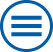## The group contributes to the following mathematical research topics of WIAS:

Systems of partial differential equations: modeling, numerical analysis and simulation

The mathematical modelling of many scientific and technological problems leads to (initial) boundary value problems with systems of partial differential equations (PDEs). [>> more]

Theory of dynamical systems

The theory of dynamical systems plays an important role in the mathematical description of time-dependent processes in various fields, such as physics and technology, biology or economics. It includes the study of systems of ordinary differential equations, partial differential equations, delay-differential equations and iterated mappings. [>> more]